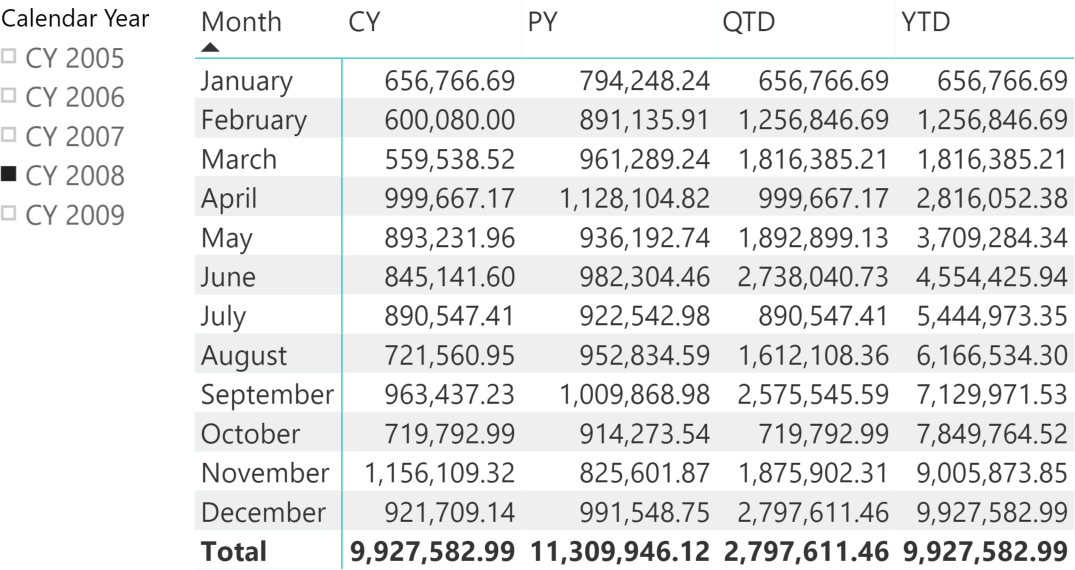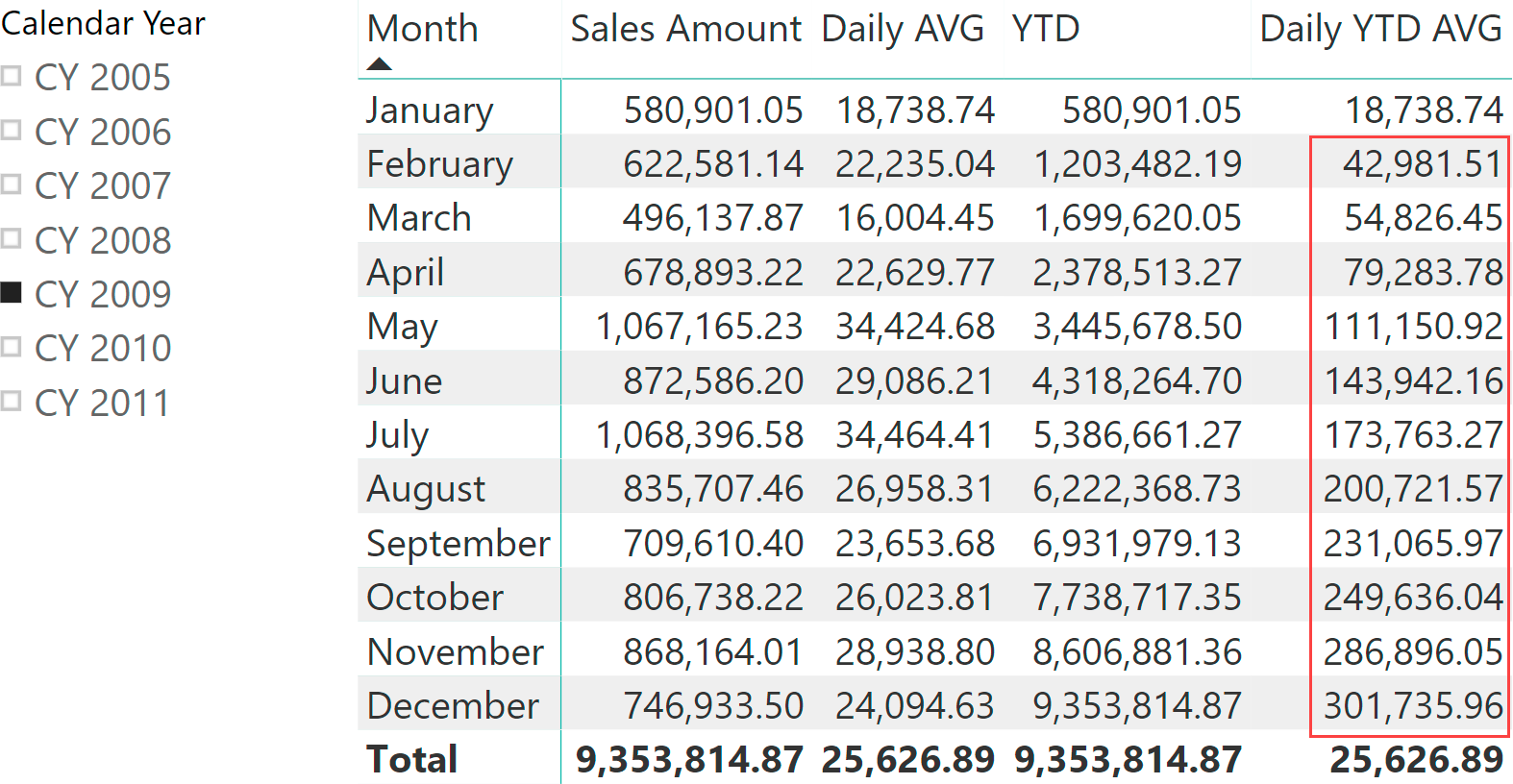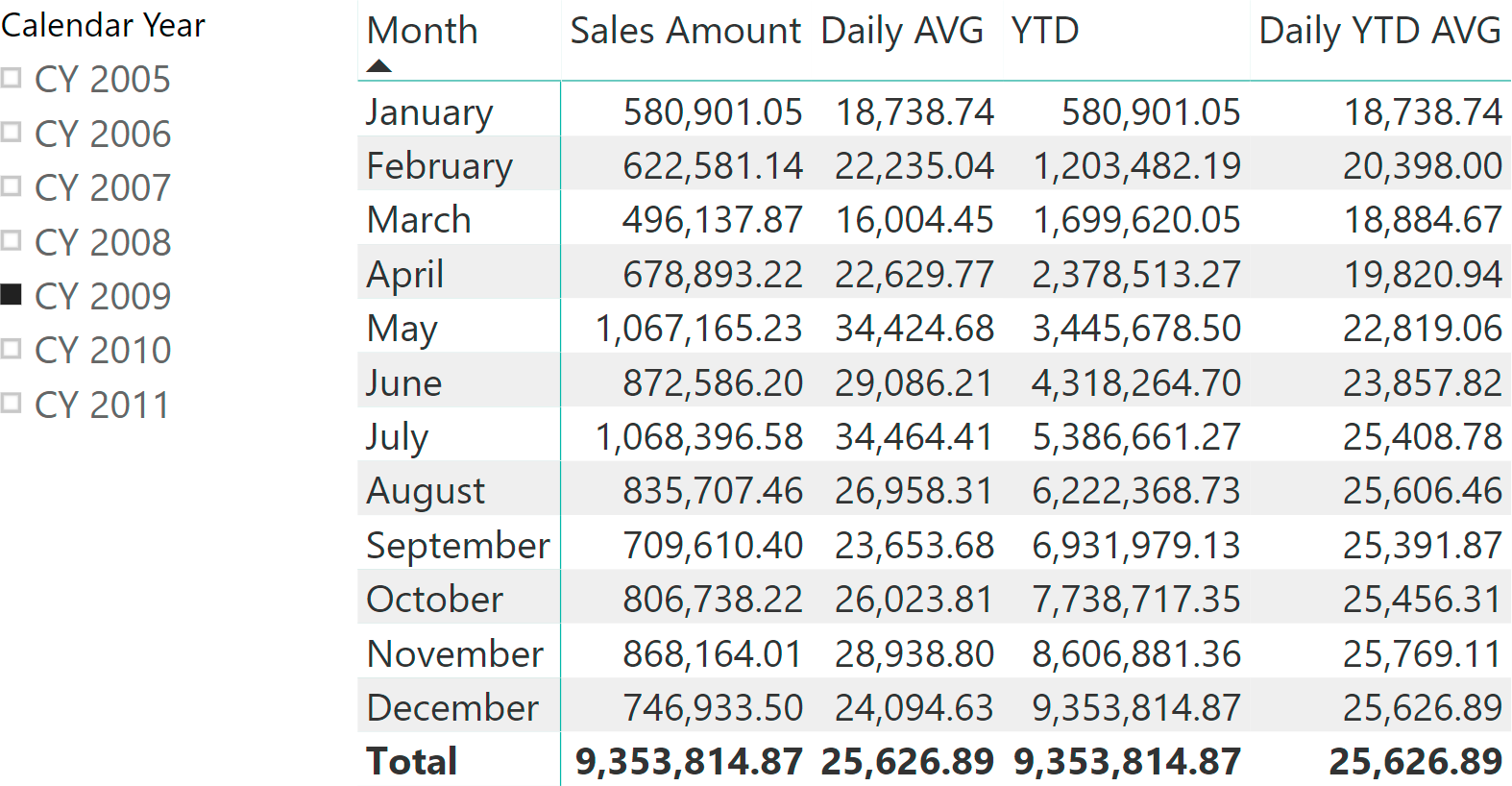It is possible to define multiple calculation groups in one same data model. Moreover, it is possible to apply multiple calculation items to the same measure. Even though each calculation group can only have one active calculation item, the presence of multiple calculation groups can activate multiple calculation items at the same time. This happens when a user uses multiple slicers over different calculation groups, or when a CALCULATE function filters calculation items in different calculation groups. For example, in the first article about calculation groups we defined two calculation groups: one to define the base measure and the other to define the time intelligence calculation to apply to the base measure. We obtained the following result, where the user selects both the time intelligence calculation and the base measure for the report.If there are multiple calculation items active in the current filter context, it is important to define which calculation item is applied first, by defining a set of precedence rules. DAX enforces this by making it mandatory to set the Precedence property in a calculation group, in models that have more than one calculation group. This article describes how to correctly set the Precedence property of a calculation group by showing several examples where the definition of the precedence changes the result of the calculations.

To prepare the demonstration, we created two different calculation groups, each one containing only one calculation item:

```-------------------------------------------------------
-- Calculation Group: 'Time Intelligence'[Time calc]
-------------------------------------------------------

--
-- Calculation Item: YTD
--
CALCULATE (
SELECTEDMEASURE (),
DATESYTD ( 'Date'[Date] )
)

-------------------------------------------------------
-- Calculation Group: 'Averages'[Averages]
-------------------------------------------------------

--
-- Calculation Item: Daily AVG
--
DIVIDE (
SELECTEDMEASURE (),
COUNTROWS ( 'Date' )
)
```

YTD is a regular year-to-date calculation, whereas Daily AVG computes the daily average by dividing the selected measure by the number of days in the filter context. Based on the two calculation items, we defined two measures:

```YTD :=
CALCULATE (
[Sales Amount],
'Time Aggregation'[Aggregation] = "YTD"
)

Daily AVG :=
CALCULATE (
[Sales Amount],
'Averages'[Averages] = "Daily AVG"
)
```

Both measures work just fine, as you can see in the following report.The scenario suddenly becomes more complex when both calculation items are used at the same time. Look at the following Daily YTD AVG measure definition:

```Daily YTD AVG :=
CALCULATE (
[Sales Amount],
'Time Intelligence'[Time calc] = "YTD",
'Averages'[Averages] = "Daily AVG"
)
```

The measure invokes both calculation items at the same time, but this raises the issue of precedence. Should the engine apply YTD first and Daily AVG later, or the other way around? In other words, which of these two expressions should be evaluated?

```--
--  YTD is applied first, and then DIVIDE
--
DIVIDE (
CALCULATE (
[Sales Amount],
DATESYTD ( 'Date'[Date] )
),
COUNTROWS ( 'Date' )
)

--
--  DIVIDE is applied first, and then YTD
--
CALCULATE (
DIVIDE (
[Sales Amount],
COUNTROWS ( 'Date' )
),
DATESYTD ( 'Date'[Date] )
)
```

It is likely that the second expression is the correct one. Nevertheless, without further information, DAX cannot choose between the two. Therefore, you must define the correct order of application of the calculation groups.

The order of application depends on the Precedence property in the two calculation groups: The calculation group with the highest value is applied first; then the other calculation groups are applied according to their Precedence value in a descending order. For example, you produce the wrong result with the following settings:

• Time Intelligence calculation group – Precedence: 0
• Averages calculation group – Precedence: 10The value of the Daily YTD AVG is clearly wrong in all the months displayed but January. Let us analyze what happened in more depth. Averages has a precedence of 10; therefore, it is applied first. The application of the Daily AVG calculation item leads to this expression corresponding to the Daily YTD AVG measure reference:

```CALCULATE (
DIVIDE (
[Sales Amount],
COUNTROWS ( 'Date' )
),
'Time Intelligence'[Time calc] = "YTD"
)
```

At this point, DAX activates the YTD calculation item from the Time Intelligence calculation group. The application of YTD rewrites the only measure reference in the formula, which is Sales Amount. Therefore, the final code corresponding to the Daily YTD AVG measure becomes the following:

```DIVIDE (
CALCULATE (
[Sales Amount],
DATESYTD ( 'Date'[Date] )
),
COUNTROWS ( 'Date' )
)
```

Consequently, the number shown is obtained by dividing the Sales Amount measure computed using the YTD calculation item, by the number of days in the displayed month. For example, the value shown in December is obtained by dividing 9,353,814,87 (YTD of Sales Amount) by 31 (the number of days in December). The number should be much lower because the YTD variation should be applied to both the numerator and the denominator of the DIVIDE function used in the Daily AVG calculation item.

To solve the issue, the YTD calculation item must be applied before Daily AVG. This way, the transformation of the filter context for the Date column occurs before the evaluation of COUNTROWS over the Date table. In order to obtain this, we modify the Precedence property of the Time Intelligence calculation group to 20, obtaining the following settings:

• Time Intelligence calculation group – Precedence: 20
• Averages calculation group – Precedence: 10

Using these settings, the Daily YTD AVG measure returns the correct values.This time, the two application steps are the following: DAX first applies the YTD calculation from the Time Intelligence calculation group, changing the expression to the following:

```CALCULATE (
CALCULATE (
[Sales Amount],
DATESYTD ( 'Date'[Date] )
),
'Averages'[Averages] = "Daily AVG"
)
```

Then, DAX applies the Daily AVG calculation item from the Averages calculation group, replacing the measure reference with the DIVIDE function and obtaining the following expression:

```CALCULATE (
DIVIDE (
[Sales Amount],
COUNTROWS ( 'Date' )
),
DATESYTD ( 'Date'[Date] )
)
```

The value displayed in December now considers 365 days in the denominator of DIVIDE, thus obtaining the correct number. Before moving further, please consider that, in this example, we followed the best practice of using calculation items with a single measure. Indeed, the first call comes from the visual of Power BI. However, one of the two calculation items rewrote the Sales Amount measure in such a way that the problem arose. In this scenario, following the best practices is not enough. It is mandatory that you understand and define the precedence of application of calculation groups very well.

All calculation items in a calculation group share the same precedence. It is impossible to define different precedence values for different calculation items within the same group.

The Precedence property is an integer value assigned to a calculation group. A higher value means a higher precedence of application; the calculation group with the higher precedence is applied first. In other words, DAX applies the calculation groups according to their Precedence value sorted in a descending order. The absolute value assigned to Precedence does not mean anything. What matters is how it compares with the Precedence of other calculation groups. There cannot be two calculation groups in a model with the same Precedence.

Because assigning different Precedence values to multiple calculation groups is mandatory, you must pay attention when making this choice at the model design stage.. Choosing the right Precedence upfront is important because changing the Precedence of a calculation group might affect the existing reports of a model already deployed in production. When you have multiple calculation groups in a model, you should always spend time verifying that the results of the calculations are the results expected with any combination of calculation items. The chances of making mistakes in the definition of the precedence values is quite high without proper testing and validation.

## Conclusions

Calculation items and calculation groups are extremely powerful. At the same time, they are quite complex if used in non-trivial scenarios. Specifically, using multiple calculation groups in the same model requires you to define the precedence of application of calculation items. Using the wrong setting for the precedence produces incorrect results which, moreover, are extremely hard to test and debug.

If you need to use multiple calculation groups in the same model, then spend time learning and understanding the details of this article before moving further with the development. It is time well spent, as it is going to save you a lot of debug time later, when the model is in production.